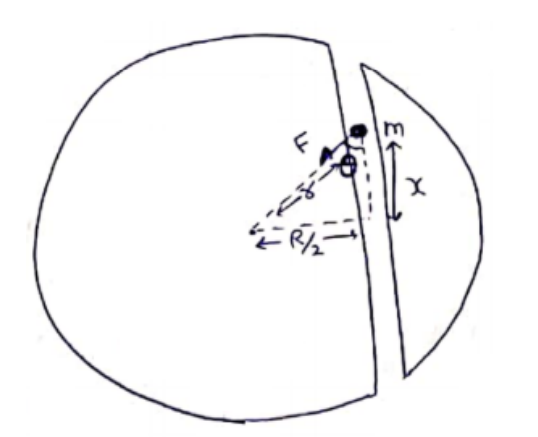# Solve the following :Question:

A tunnel is dug along a chord of the earth at a perpendicular distance $R / 2$ from the earth's center. The wall of the tunnel may be assumed to be frictionless. Find the force exerted by the wall on a particle of mass $m$ when it is at a distance $x$ from the center of the tunnel.

Solution:Distance of the particle from earth's center

$r=\sqrt{\left(\frac{R}{2}\right)^{2}+x^{2}}$

Force on the particle=mass ${ }^{X}$ gravitational field intensity

$F=m\left(\frac{G M r}{R^{3}}\right)$

$G=\frac{G M m}{R^{3}} r$

Force exerted by wall on particle=Force exerted by particle on wall

$=F \sin \theta$

$=\frac{G M m r}{R^{3}}\left(\frac{R}{2 r}\right)$

$=\frac{G M m}{2 R^{2}}$